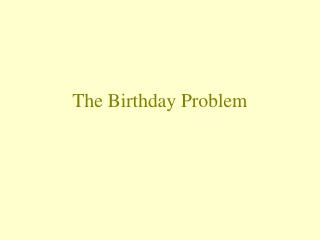DownloadDownload PresentationThe Birthday Problem

The Birthday Problem

Télécharger la présentationThe Birthday Problem

- - - - - - - - - - - - - - - - - - - - - - - - - - - E N D - - - - - - - - - - - - - - - - - - - - - - - - - - -
Presentation Transcript

1. The Birthday Problem

2. The Problem • In a group of 50 students, what is the probability that at least two students share the same birthday?

3. Assumptions • Only 365 days each year. • Birthdays are evenly distributed throughout the year, so that each day of the year has an equal chance of being someone’s birthday. Note: The accuracy of the probability calculations that we do here depends on whether or not these assumptions are valid.

4. Take group of 5 people…. Let A = event no one in group shares same birthday. Then AC = event at least 2 people share same birthday. Assuming independence and using classical approach: P(A) = 365/365 × 364/365 × 363/365 × 362/365 × 361/365 = 0.973 So, then P(AC) = 1 - 0.973 = 0.027 That is, about a 3% chance that in a group of 5 people at least two people share the same birthday.

5. Take group of 23 people…. Let A = event no one in group shares same birthday. Then AC = event at least 2 people share same birthday. Assuming independence and using classical approach: P(A) = 365/365 × 364/365 × … × 343/365 = 0.493 So, then P(AC) = 1 - 0.493 = 0.507 That is, about a 51% chance that in a group of 23 people at least two people share the same birthday.

6. Take group of 50 people…. Let A = event no one in group shares same birthday. Then AC = event at least 2 people share same birthday. Assuming independence and using classical approach: P(A) = 365/365 × 364/365 × … × 316/365 = 0.03 P(AC) = 1 - 0.03 = 0.97 That is, about a 97% chance that in a group of 50 people at least two people share the same birthday.